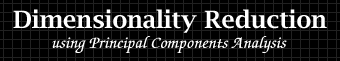by François LabelleSo you have `n` objects, and for each of them you performed `m` measurements, giving you `n` points in `m` dimensions. Because the measurements are not necessarily independent, the data is likely to be shaped like a galaxy, that is, the set of points almost lies in some 2D-plane. This page demonstrates how to rotate multidimensional data so that the best plane-fit is your screen. As you'll see, this can be an excellent visualization tool.

## Introductory example: planets

If you have only 2 measurements for your objects, then a good way to visualize the data is to plot the data in the plane, using the two measurements as axes.

For instance, two important features of the planets in our solar system are:
 1.2. the distance to the Sunthe equatorial diameter

Making the data plot will give you a nice representation with obvious properties:

• close points mean similar planets
• far apart points mean dissimilar planets
• convex hull points mean "extreme" planets
Now suppose that there is another planet feature that you find equally important:
 3 the density

Since 3D graph-paper is rather hard to find, the question is: can we still have a 2D-plot of the data such that the properties above still hold? (i.e. close points in the 2D-plot mean similar planets for all 3 features, etc.) In this particular example the answer is yes. There exists a 2D-projection that keeps 97% of the variance of the 3D-data.

Below you can see the distance/diameter log-plot of the data (which contains no information on density). Click on the "rotate!" button to see the best 2D-projection: a diagram that is meaningful for distance, diameter and density.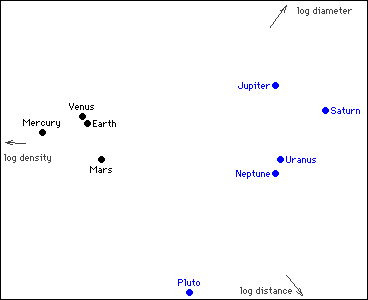Your browser does not support Java applets. Here's a static image instead.variance shown: 97%

see the data file

A glance at the rotated data confirms that Venus is Earth's twin, that Uranus and Neptune are also similar, and that Pluto is a rather strange object. That's the beauty of these diagrams!

## What are we doing exactly?

technical section

### Obtaining the data

• Let `n` be the number of objects.
• Let `m` be the number of measurements.
• Let `xij` be the value of measurement `i` performed on object `j`.

### Preparing the data

Sometimes when the order of magnitude of measurement `i` varies too much, it makes sense to replace the measurement by its logarithm:
 `xij <- log(xij)` `for j = 1..n`

Then the measurements are centered about their means:
 `xij <- xij - mi` `for i = 1..m, j = 1..n` where: `mi = (xi1 + xi2 + .. + xin)/n`

If the measurements don't have the same unit, it makes sense to standardize them:
 `xij <- xij/si` `for i = 1..m, j = 1..n` where: `si = sqrt((xi12 + xi22 + .. + xin2)/n)`

Finally, if some measurement `i` is considered more important, it can be weighted by an appropriate factor:
 `xij <- wi * xij` `for j = 1..n`

### Rotating the data

We wish to rotate the data so that it lies as flat as possible in the first 2 dimensions of the space.

• Let `U` be any orthogonal `m x m` matrix.
• Let `xj = [x1j x2j .. xmj]T` denote the measurement vector of object `j`.
• For each object `j`, let `yj = UT * xj` be its rotated measurement vector.
• For each axis `i`, let `vi = (yi12 + yi22 + .. + yin2)/n` be the variance in this axis.
We wish to maximize the variance in the first 2 axes. i.e.:

• Find `U` such that `v1 + v2` is maximum.
As it turns out, we can maximize `v1` first, keep it fixed and then maximize `v2`.

If we do so, then `u1` (the first column of the matrix `U`) is called the first principal component, and `u2` is called the second principal component. (These two vectors span the best plane-fit that we are looking for.)

In general we can continue: keep the variance of the first 2 axes fixed and maximize `v3`, etc... Then the `ith` principal component is given by `ui`, and its contribution to the total variance of the data is `vi`.

### Computing the rotation matrix `U`

There are many ways of computing the matrix `U` and they are all pretty involved. Let's just say that in our case, the applet starts with the identity matrix `I`, and applies `2 x 2` rotations (each of them making a local improvement) until it gets `U`.

### Other definitions of plane-fitting

When we are using the first two principal components to define a best plane-fit, we are implicitly defining the best plane-fit to be the one that minimizes the sum of the squares of the distances of the points to that plane.

This is not the only possible definition, and you may want to see this page on Line and Plane Fitting.

## Learning to use the applet

### Another example: countries

Here we would like to get a 2D-diagram to classify some countries based on the following 3 features:
 1.2.3. populationaverage incomearea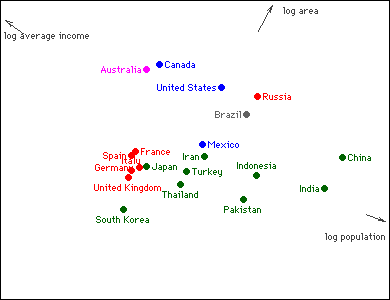Your browser does not support Java applets. Here's a static image instead.variance shown: 88%

see the data file

### Description of the applet

The applet starts by plotting the data along the first two measurements. On the right of the image, you have the following commands and displays:

 rotate!: To show the principal-components-finding algorithm in action. See how it tries to spread the points by a succession of rotations. x-axis, y-axis: To decide which axes to see. (PC_1, PC_2,... are the principal components). variance shown: Displays the quality measure of the displayed orientation (it is maximal when PC_1 and PC_2 are the axes). show labels, show axes: To clutter or unclutter the picture at will. show error bars: To show how far each point is from the plane-fit. Equivalently, the algorithm tries to minimize the sum of the squares of the lengths of these bars.

## Application to image analysis

### Image processing

In satellite imaging, it's easy to get overwhelmed by measurements by imaging at various wavelengths and by repeating the measurements in different conditions. Principal components analysis can be used to reduce the dimensionality of the data to 3 (to get a red-green-blue image), or to analyze subtle variations by displaying less important components. The setup is:

 objects: pixels measurements: intensities at various wavelengths, in different seasons,...

### Image classification

A different situation is when you have many images and want to classify them. The brute-force approach is to declare that every pixel is a measurement. Principal components analysis will be useful in cases where similarity can be measured pixel-wise. Such a case is face recognition when the images are controlled (same size, same orientation, same lighting conditions). The setup is:

 objects: faces measurements: pixels of the image of the face (the dimensionality is very high)

### Example: the letters of the alphabet

To demonstrate image classification, we will use something simpler. All 26 letters of the alphabet have an acceptable representation on a `5x5` grid as follows: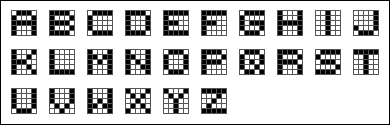This gives us 25 measurements (0-1 valued) for each letter. Click on the "rotate!" button below to reduce this 25-dimensional space to 2 dimensions: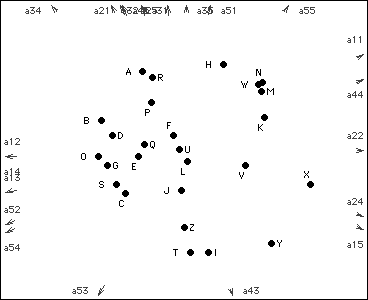Your browser does not support Java applets. Here's a static image instead.variance shown: 44%

see the data file

The variance shown is low (44%), but it's actually very good for 2 dimensions out of 25. We can spot some pairs of similar letters like `A` and `R`. It's also fun to see pairs that are far apart: `H` and `I` cannot be confused, neither can `X` and `O` (hence their use in the tic-tac-toe game).

In this application, the principal components can be viewed as filters that are applied to the bitmap to extract a feature.

Below are images of some of the filters. White is neutral, blue means a positive contribution and red means a negative contribution.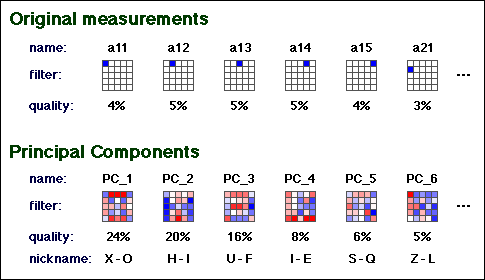## Permuting the roles of objects and measurements

What happens if we make a mistake and enter the objects as columns instead of as rows? This "mistake" is actually quite an interesting thing to do. Now we have one point per measurement, and we're trying to map the measurements to find out which ones are positively correlated (are giving similar values when applied to our set of objects).

If making a measurement is expensive, then this trick can be used to spot and eliminate redundant measurements. This is a broad topic and you may want to see this page on Feature Selection.

Here's the same data on letters of the alphabet, but with objects and measurements permuted. Click on the "rotate!" button!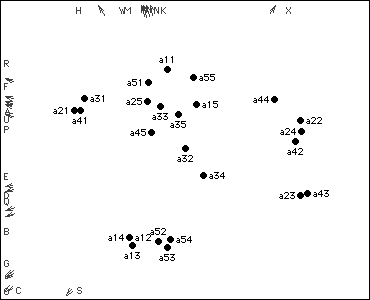Your browser does not support Java applets. Here's a static image instead.variance shown: 46%

see the data file

The two closest points on the best-fit-plot are `a12` and `a14` (they overlap), and indeed, `a12 == a14` for every letter.

The two furthest points on the best-fit-plot are `a21` and `a43`, and indeed, `a21 != a43` for every letter except `J`, `X` and `Z`. In fact these two points are so far apart that `a21` and `a43` become redundant: one is practically the opposite of the other!

 This page is presented to Godfried Toussaint as a Web project for the Pattern Recognition course 308-644B. Other student projects can be found here.
 page created: May 6, 1998 last updated: June 7, 2014